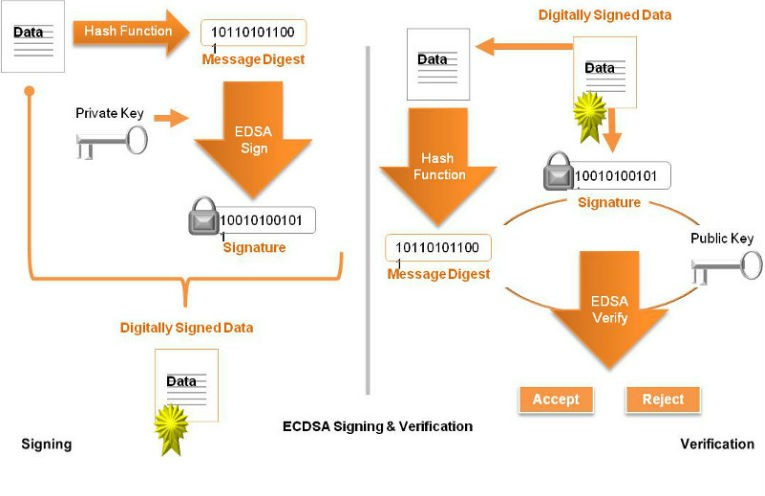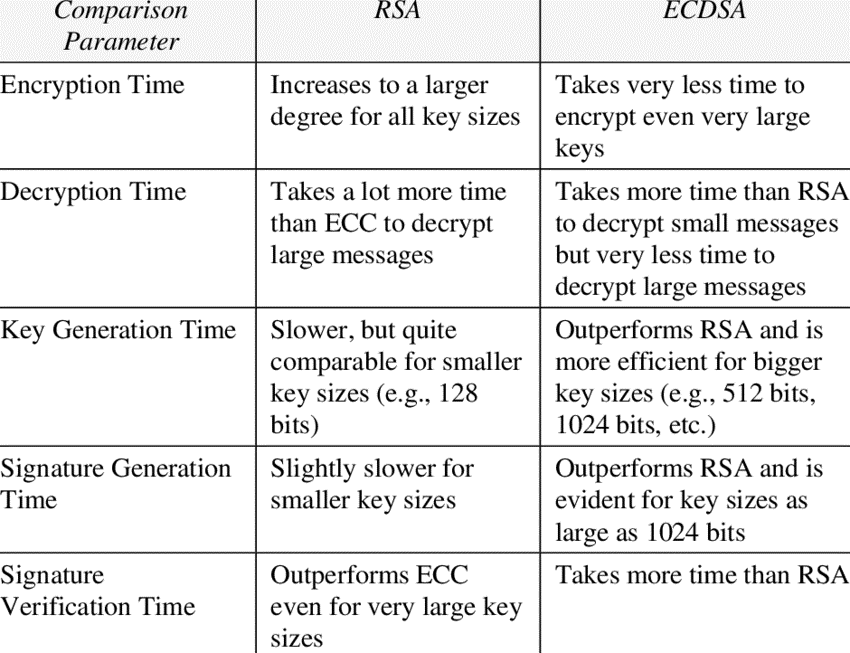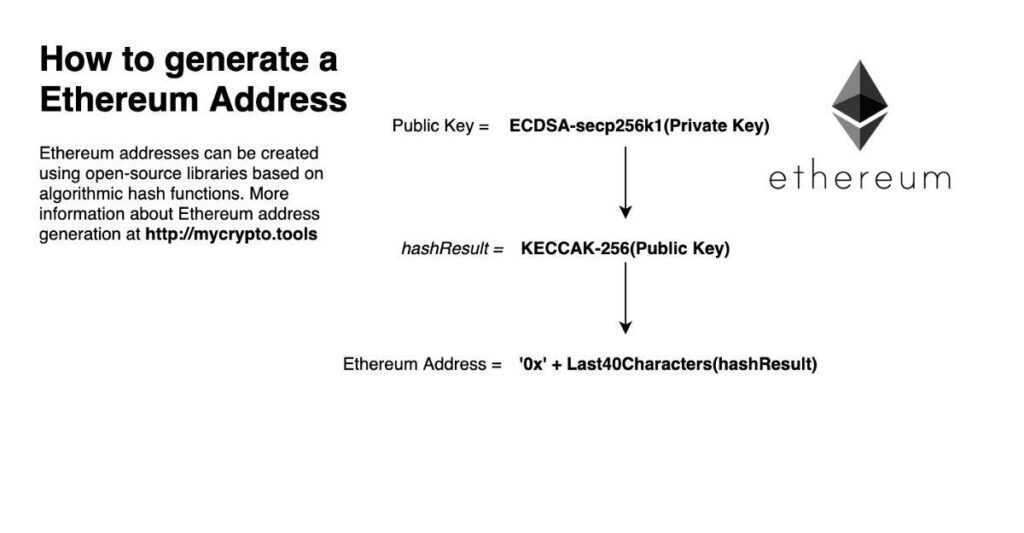• PRODUCTS

SOLUTIONS

• Blog
• Contact
What Is the Elliptic Curve Digital Signature Algorithm (ECDSA)?

DATE:

July 19 2023

AUTHOR:

# What Is the Elliptic Curve Digital Signature Algorithm (ECDSA)?

The Elliptic Curve Digital Signature Algorithm (ECDSA) is a widely used cryptographic algorithm for generating digital signatures in PKI (public key infrastructure). It offers strong security and efficient computation, making it suitable for various applications. ECDSA relies on elliptic curve mathematics to provide a high level of protection against unauthorized access and tampering. By leveraging smaller key sizes compared to traditional algorithms, ECDSA facilitates faster processing times and reduced storage requirements.

## What Is ECDSA?

ECDSA (Elliptic Curve Digital Signature Algorithm) is a public key algorithm used to build and verify an electronic digital signature using elliptic curve cryptography.

The algorithm is quite popular in the field of electronic digital signatures due to the complexity of the task on which the calculation of a private key from a public key is based. ECDSA has been adopted by various organizations as a standard. The algorithm consists of four parts: generation of basic parameters, generation of a key pair, creation, and verification of a digital signature. In general, it is considered sufficiently secure (for the appropriate levels of cryptographic strength) and also has implementations in many cryptographic libraries.

In 1991, DSA was developed by the National Institute of Standards and Technology (NIST) based on the idea of ​​using the discrete logarithm problem. Shortly thereafter, NIST requested public comments on its proposal for digital signature schemes. Inspired by this idea, Scott Vanstone in his article “Responses to NIST’s proposal” proposed an analog of the digital signature algorithm using elliptic curve cryptography (ECDSA).

In the period from 1998-2000. ECDSA has been adopted by various organizations as a standard (ISO 14888-3, ANSI X9.62, IEEE 1363-2000, FIPS 186-2).

## Digital Signature of ECDSA

For practical application, ECDSA imposes restrictions on the fields in which elliptic curves are defined. For simplicity, consider the case of implementing algorithms, when Fq is a simple finite field, (for other fields it is similar), then our elliptic equation takes the form y^(2)=x^(3)+ax+b.

In order to avoid known attacks based on the discrete logarithm problem in the group of points of an elliptic curve, it is necessary that the number of points of the elliptic curve E is divisible by a sufficiently large prime number n. The ANSI X9.62 standard requires n > 2^(160). The following algorithm is proposed:

Input: Order of field q, field representation indicator FR for Fq, L – security level: 160 ⩽ L ⩽ [log2q] and 2^L ⩾4*q^(1/2)

Conclusion: The main parameters of the elliptic curve D = (q, FR, a, b, G, n, h).

Step 1. Select randomly verified items a,b ∈ Fq satisfying the condition 4a^3+27b^2≢ 0 mod q.

Step 2. N:=#E(Fq), the order of the curve can be calculated using the SEA algorithm.

Step 3. Check that N mod n = 0 for a large prime n ⩾ 2^L. If not, then go to step 1.

Step 4. Check that q^(k)-1 mod n =0 ∀ k ∈ [1, 20]. If not, then go to step 1.

Step 5. Check that n ≠ q. If not, then go to step 1.

Step 6. ℎ:= N/n

Step 7. Choose an arbitrary point G’ ∈ E(Fq) and set G := hG’. Repeat until G ≠ O, where O is a point at infinity

Step 8. Return (q, FR, a, b, G, n, h)

Random verification algorithms guarantee that the elliptic curve over a finite field was generated completely randomly.

## Key Generation

We will consider the exchange of messages between Alice and Bob. Previously, using the algorithm for generating the main parameters, Alice obtains her main parameters of the elliptic curve. Using the following sequence of actions, Alice will generate a public and private key.

Input: Basic parameters of the elliptic curve D.

Output: Public key – Q, private key – d.

Step 1. Choose a random or pseudo-random integer d ∈ [1,n-1].

Step 2. Calculate the coordinates of the point on the elliptic curve Q=dG.

Step 3. Return (Q,d).

## ECDSA Sign

Alice, who has the main parameters of the curve D and the private key d, wants to sign the message m, for this, she must generate a signature (r,s).

In the following, H denotes a cryptographic hash function whose output message hash has a bit length of at most n (if this condition is not met, then the output value of H may be truncated). It is assumed that we are working with the function output already converted to an integer.

Input: Basic elliptic curve parameters D, private key d, message m.

Output: Signature (r,s).

Step 1. Choose a random or pseudo-random integer k ∈ [1, n-1].

Step 2. Calculate the coordinates of the point kG = (x1, y1).

Step 3 Calculate r = x1 mod (n). If r = 0 then go to step 1.

Step 4 Calculate e =H(m).

Step 5 Calculate s= k^(-1) * (e+dr) mod n. If s=0 then go to step 1.

Step 6. Return (r,s).

The ECDSA algorithm computes the public key from the signature, but this process creates ambiguity by returning zero, one, or two points on the elliptic curve representing the public key.

By introducing an additional component v to the signature, Extended ECDSA addresses this concern. The signature is represented as {r, s, v}, enabling a more reliable computation of the public key. As a result, Extended ECDSAeliminates ambiguity.

## ECDSA Verify Signature

To verify Alice’s signature (r, s) of message m, Bob receives an authentic copy of her basic curve parameters D and their associated signer’s public key Q:

Input: Basic parameters of the elliptic curve D, public key Q, message m, signature (r,s).

Output: The decision to accept or reject the signature.

Step 1. Check that r,s are integers belonging to [1, n − 1]. If any validation fails, then return “Reject”.

Step 2 Calculate e = H(m).

Step 3 Calculate w = s^(-1) mod n.

Step 4 Calculate u1 = ew mod n and u2 = rw mod n.

Step 5. Calculate the coordinates of the point X=(x2, y2) =u1G+u2Q.

Step 6. If X = O, then return “Reject”. Otherwise, compute v = x2 mod n.

Step 7. If v = r, then return “Accept”, otherwise “Reject”

## How Does It Work?In this example, only meaningful computational steps in the algorithms will be described, assuming that all checks can be made without a textual description.

1. Using the algorithm for generating basic parameters, we obtain the following values: p=114973, elliptic curve E: y^(2)=x^(3)-3x+69424, and base point G=(11570,42257) with order n= 114467.
2. Let’s generate a pair of keys, in accordance with the algorithm for generating a key pair:
• Step 1. Choose d = 86109 ∈ [1, 114466].
• Step 2. Calculate the coordinates of the point Q = dG = (6345, 28549).

1. Using the digital signature generation algorithm, we will sign the message given in the form of text m = worldof with the value of the hash function e = H(m) = 1789679805.
• Step 1. Choose k=84430 ∈ [1,114466].
• Step 2. Calculate the coordinates of the point kG = (x1, y1) = (31167, 10585).
• Step 3 Calculate r = x1 mod n =31167 mod 14973 = 31167.
• Step 4 Calculate s = k^(-1)*(e+dr) mod n = 84430^(-1) (1789679805+86109*31167) mod 14973 = 82722.

1. Check the authenticity of the signature (r, s) for the message m using the digital signature verification algorithm.
• Step 1 Calculate w = s^(-1) mod n = 82722^(-1) mod 14973 = 83035.
• Step 2. Calculate u1 = ew mod n = 1789679805 * 83035 mod 14973 = 71001 and u(2) = rw mod n = 31167*83035 mod 14973 = 81909.
• Step 3. Calculate the coordinates of the point X = u1G + u2Q = (66931, 53304) + (88970, 41780) = (31167, 31627).
• Step 4. Calculate v = x2 mod n = 31167 mod 14973 = 31167.
• Step 5. Check v = r = 31167. Accept the signature.The strength of the encryption algorithm is based on the problem of the discrete logarithm in a group of points on an elliptic curve. Unlike the simple discrete logarithm problem and the integer factorization problem, there is no subexponential algorithm for the discrete logarithm problem on the group of points of an elliptic curve. For this reason, the “power per key bit” is significantly higher in an algorithm that uses elliptic curves.

This means that much smaller parameters can be used in elliptic curve cryptography than in other public key cryptography systems such as RSA but with an equivalent level of security. For example, the bit size of keys: a 160-bit key would be equivalent to keys with a 1024-bit modulus in RSA with a comparable level of security against known attacks. Benefits gained from smaller parameter sizes include algorithm execution speed, efficient use of energy, and bandwidth memory. They are especially important for applications on devices with limited capabilities, such as smart cards.

## The Benefits and Drawbacks to Using ECDSA

ECDSA, or Elliptic Curve Digital Signature Algorithm, offers several benefits and drawbacks in the realm of cryptographic security. One of the primary advantages of ECDSA is its strong security. The algorithm utilizes elliptic curve mathematics, making it resistant to brute force attacks and providing a high level of protection against unauthorized access and tampering. Moreover, ECDSA offers efficient computation and shorter key lengths compared to traditional RSA-based algorithms, resulting in faster processing times and reduced storage requirements.

Another benefit of ECDSA is its suitability for resource-constrained environments, such as mobile devices or embedded systems. Its ability to generate small key sizes while maintaining robust security makes it ideal for scenarios with limited computational power or memory.

However, ECDSA does have its drawbacks. One of the main concerns is the need for trusted elliptic curve parameters. The security of ECDSA relies heavily on the selection of appropriate curves, and any weaknesses or backdoors in the chosen parameters could compromise the entire system. Therefore, careful implementation and verification of the elliptic curve parameters are crucial.

Additionally, ECDSA may suffer from slower verification speeds compared to other signature algorithms, especially as the key sizes increase. Verifying ECDSA signatures can be computationally intensive, which may impact performance in scenarios that require rapid signature verification.

## Limitations of ECDSA

One of the significant limitations is the lack of forward secrecy. With ECDSA, if an adversary obtains a private key, they can retroactively calculate all past signatures associated with that key. This implies that if an ECDSA private keyis compromised, all previous signatures become vulnerable, potentially jeopardizing the integrity and authenticity of previously verified data.

Another limitation lies in the potential vulnerability to side-channel attacks. Side-channel attacks exploit information leaked during the execution of cryptographic operations, such as timing or power consumption. While ECDSA itself is theoretically secure, its implementation on physical devices may be susceptible to side-channel attacks, compromising the confidentiality of the private key.

Additionally, ECDSA relies on the presence of trusted random number generation during the signing process. If the random number generator used is flawed or compromised, it can lead to predictable or weak signatures, undermining the security of the algorithm. The choice of elliptic curves for ECDSA is critical. If an insecure or weak curve is used, it can introduce vulnerabilities and potentially weaken the overall security of the system

## Where Can ECDSA Be Implemented?

ECDSA is implemented in such cryptographic libraries as OpenSSL, Cryptlib, Crypto++, GnuTLS protocol implementations, and CryptoAPI application programming interface. There are many other software implementations of the elliptic curve digital signature algorithm, most of which are mainly focused on one application, for example, a fast implementation for one specific finite field.

In Ethereum, extended ECDSA is utilized for transaction signing. Due to the limited bandwidth and storage capacity in a blockchain environment, extended ECDSA proves advantageous as it eliminates the need to transmit or store public keys separately. Instead, the public keys can be derived from the signature itself.## Conclusion

Elliptic Curve Digital Signature Algorithm (ECDSA) stands as a robust and efficient cryptographic algorithm for generating digital signatures in PKI (public key infrastructure). Its utilization of elliptic curve mathematics provides strong security, making it resistant to brute force attacks and tampering. With smaller key sizes and faster processing times, ECDSA offers practical benefits in terms of efficiency and resource utilization. Helenix develops cryptographic systems for a wide variety of organizational needs. Learn more about our expertise in the Custom Development section.

## FAQ

ECDSA offers advantages like smaller key sizes and faster computation, making it more efficient for resource-constrained environments. However, both algorithms have their strengths and weaknesses, and the choice depends on specific requirements.

RSA is based on integer factorization, while ECDSA relies on elliptic curve mathematics. ECDSA generally offers smaller key sizes, faster computation, and efficient resource utilization compared to RSA.

One example of ECDSA is its usage in cryptocurrencies like Bitcoin. ECDSA is employed to generate and verify digital signatures for transactions, ensuring their integrity and authenticity.

ECDSA is an algorithm that utilizes the principles of elliptic curve cryptography (ECC). ECC is a broader cryptographic framework, while ECDSA specifically refers to the digital signature algorithm based on ECC.

An ECDSA certificate, also known as an EC certificate, is a digital certificate that uses ECDSA to secure the certificate’s integrity and authenticity. It contains a public key, signature, and identifying information.